Related Articles

# Create Array of Zeros in MATLAB

• Last Updated : 04 Jul, 2021

MATLAB generally stores its variables in matrix forms, also in array and vector form. Sometimes, we often need a matrix(or array or vector) of zero(s) for some specific operations. We can create a matrix of zero(s) manually or with the help of the in-built function of MATLAB. The in-built function that is used to create an array or matrix of zero(s) is called the zeros() function. We generally prefer this in-built function for the ease of the task rather than create an array of zeros manually. The following part contains the two methods of creating an array or matrix of zeros.

### 1. Creating an array of zeros manually

If we want to create an array of zeros we can simply do that manually by using the following code:

Example:

## Matlab

 `% MATLAB Code for  create ``% an array of zeros ``X = [0 0 0 0 0];``disp(X)`

It is basically a row vector of size 1X5 as well as an array of 5 zeros.

Output:Output Screenshot

Creating a column vector manually: If we want to create a column vector we can just use the following code:

## Matlab

 `% MATLAB code to create a ``% column vector withe zero's``X = [0; 0; 0; 0; 0]`

It basically creates a column vector of size 5X1.

Output: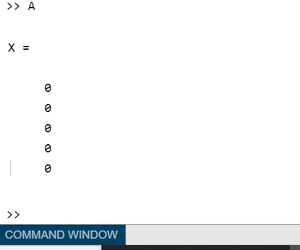Output Screenshot

Creating a (n x m) matrix of zeros: In this section, we are going to create an (n x m)  dimensional matrix. For example, let n = 3 and m = 4. Basically, the following line of code will generate a matrix with 3 rows and 4 columns.

## Matlab

 `% MATLAB code for create an ``% (n x m)  dimensional matrix``X = [0 0 0 0; 0 0 0 0; 0 0 0 0]`

Output: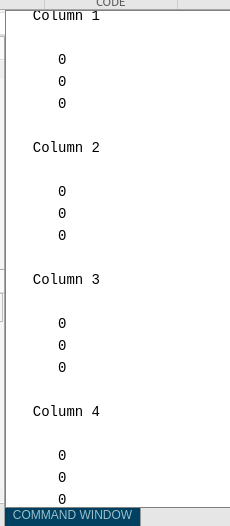Output Screenshot

#### 2. Creating array of zeros using in-built function (zeros())

There are several matrices and arrays that we can create using the zeros() function. We are going to describe each of them elaborately in the following section:

Creating scalar zero

Syntax: variable_name = zeros

Return value: In this case, the return value is only scalar zero(‘0’).

Example:

## Matlab

 `% MATLAB Code for Creating scalar zero``X = zeros`

Output: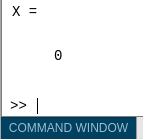Output Screenshot

Creating a (n x n) matrix of zeros

syntax: matrix = zeros(n)  // Here n is the size of matrix.

Return value: zeros(n) function returns a (n x n) matrix of zeros:

Input Arguments:

1. Size of square matrix, specified as an integer value.
2. If n is 0 then it returns an empty matrix.
3. If n is negative, it also returns an empty matrix.

Example:

## Matlab

 `% MATLAB code for Creating a (n x n) matrix of zeros``matrix = zeros(3)`

This code returns a 3 x 3 matrix of zeros.

Output: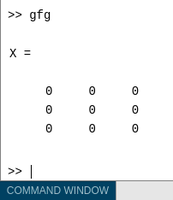Output Screenshot

Creating a (sz1 by sz2 by-…….-szn) array of zeros

syntax:  matrix = zeros(sz1, sz2,…….,szn)

Return value:  This function returns an sz1-by-…-by-szN array of zeros where sz1,…,szN indicate the size of each dimension. For example, zeros(2, 3, 4) returns a 2 X 3 X 4 matrix of zeros.

Input Arguments:

1. all the input arguments are specified as an integer value.
2. If any of the argument is 0, it returns an empty matrix.
3. If any of the argument is negative then it is treated as 0.
4. Beyond the second dimension, zeros() ignores trailing dimensions with a size of 1. For example, zeros(4,1,1, 1) produces a 4-by-1 vector of zeros.

Example:

## Matlab

 `% MATLAB Code for 3-d matrix of zeros``matrix = zeros(2, 3, 4)`

The above code creates a 2-by-3-by-4 array of zeros.

Creating a matrix of a specific size

Syntax: matrix = zeros(sz) // Here sz is the dimension of the matrix in the form [m n].

Return value:  It returns an array of zeros where size vector sz defines size(matrix). For example, zeros([2 3]) returns a 2-by-3 matrix.

Input Arguments:

Size of each dimension, specified as a row vector of integer values. The rest of the characteristics of this syntax are as same as the previous one.

Example:

## Matlab

 `% MATLAB Code for clone the size of ``% another matrix``matrix = zeros([2 3])`

or we can also clone the size of another matrix like the following:

## Matlab

 `% MATLAB code for clone the another``% matrix in different way``A = ones(2, 3);``matrix = zeros(size(A))`

The above code basically returns a matrix of 2 X 3 with zeros as every element.

Output: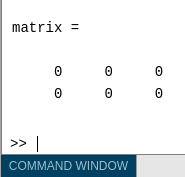Output Screenshot

Creating specified data types of zeros

syntax: matrix = zeros(___,typename)  // Here the first argument may be any of the previous types.

Return value:  It returns an array of zeros of  the specified data type named typename.

Input Arguments:

Data type (class) to create, specified as ‘double’, ‘single’, ‘logical’,’int8′, ‘uint8’, ‘int16’, ‘uint16’, ‘int32’, ‘uint32’, ‘int64’, ‘uint64’, or the name of another class that provides zeros support.

Example:

## Matlab

 `% Code``A = ones(2, 3);``matrix = zeros(size(A), ``'uint32'``)`

This code creates a 2-by-3 matrix of datatype ‘uint32’.

Output: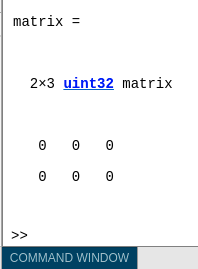Output Screenshot

Creating matrix using a prototype of an array

syntax:  matrix = zeros(___,’like’,p) // Here the first argument may be any of the previous types.

Return value:  It returns an array of zeros like p; i.e, of the same data type (class), sparsity, and complexity (real or complex) as p. The user can specify typename or ‘like’, but not both.

Input Arguments:

Prototype of array to create, specified as an array.

Example

## Matlab

 `% MATLAB Code for clone complexity``% of an array``p = [2+2i 13i];``X = zeros(``'like'``,p)`

Output:Example:

## Matlab

 `% MATLAB code for clone Size and``% Data Type from Existing Array ``p = uint8([4 5 6; 14 15 16; 12 13 14]);``matrix = zeros(size(p),``'like'``,p)`

Output: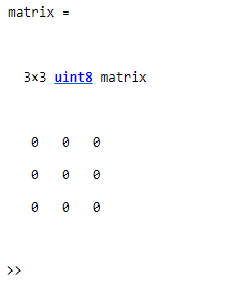My Personal Notes arrow_drop_up